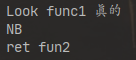# 浅谈JVM语言之函数式编程

## 见微知著。

Posted by YSY on June 10, 2020

## Java中的函数式编程

### 一些基本特点总结

• 相比平常的指令式编程，函数式编程更在乎执行结果而非过程；
• 函数是一等公民，可以像普通的数值、引用等变量一样赋值、作为参数传递、作为返回值；
• 函数是纯函数，即函数不能产生副作用，如不能修改全局变量等，固定的输入就映射固定的输出。

### 简单示意一下

``````// 定义一个函数g，并赋值给f
f = g(x, y) = x + y
// 写一个方法，函数作为参数传递
printF(g) {
print(g(1, 2))
}
// 调用方法
printF(f) // 打印结果3
// 作为返回值
getF() {
return f
}
``````

### Java函数式编程

``````Runnable f = ()-> {
// do something
}
void testF(Runnable r, int i) {
print(i);
r.run();
}
testF(f, 1);
// 看这个是不是有点函数式编程的影子了，其实Runnable接口的设计在Java 8之前就有了，还是很有远见的，在此可以把f看成一个无参无返回值的函数，也算是低配版的函数式编程嘛~
``````

### Groovy函数式编程

``````def func1 = { msg1 ->
println "Look func1 \$msg1"

def fun2 = { msg2 ->
println msg2
"ret fun2" // 在闭包中最后一行值将直接作为返回结果，加不加return都可
}
return fun2
// 等效简化代码
//    return { msg2 ->
//        println msg2
//        "ret fun2"
//    }
}

println func1('真的')('NB')
``````## 柯里化理论基础

Java8柯里化示例：

``````import java.util.function.Function;
import java.util.function.IntFunction;
import java.util.function.IntUnaryOperator;

public class Curry {
private static final Function<Integer, Function<Integer, Function<Integer, Integer>>> CURRYING_1 =
x -> y -> z -> (x + y) * z;
private static final IntFunction<IntFunction<IntUnaryOperator>> CURRYING_2 =
x -> y -> z -> (x + y) * z;
private static final TriFunction<Integer, Integer, Integer, Integer> CURRYING_3 =
(x, y, z) -> (x + y) * z;

public static void main(String[] args) {
System.out.println(CURRYING_1.apply(1).apply(2).apply(3));
System.out.println(CURRYING_2.apply(1).apply(2).applyAsInt(3));
System.out.println(CURRYING_3.apply(1, 2, 3));
}

@FunctionalInterface
public interface TriFunction<U, T, S, R> {
R apply(U u, T t, S s);
}
}
``````

## 低版本Java兼容实践

``````// build.gradle
android {
compileOptions {
sourceCompatibility JavaVersion.VERSION_1_8
targetCompatibility JavaVersion.VERSION_1_8
}
}
``````

``````// 比如Consumer就是一种只接受一个输入，而没有输出的特殊函数
public interface Consumer<T> {
void accept(T t);
}

// 为通知构建，创建一个PendingIntent
public static PendingIntent createActivityI(int type, Consumer<Intent> consumer) {
Context appCtx = MyApp.getContext(); // 获取App全局Context
Intent intent = new Intent();
if (consumer != null) {
consumer.accept(intent);
}
return PendingIntent.getActivity(appCtx, type, intent, PendingIntent.FLAG_UPDATE_CURRENT);
}
// 外部调用
PendingIntent clickI = createActivityI(INTENT_TYPE_TEST, intent -> {
intent.setData(xxx);
intent.putExtra(xxx);
// ...对intent对象各种操作，无需关心内部是如何初始化的（实例化），我们只是外部消费者（consumer）
});
``````

## 请勿滥用